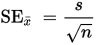Help Center
1. Help Center
2. Analyze
3. SiteSpect Reports

# Understanding the Confidence Plot

The Confidence Plot (Plot) on the Performance Matrix is a visual representation of the Confidence Intervals1. For each Variation Group, for a given Metric, it graphically shows the Confidence Interval for the Variation Group when compared with the Control Group.

The Plot uses 80% Confidence. For each Variation Group (for a given Metric), the interval is defined as:

Avg +/- (1.281552 * SE)

Avg is the average value of the Metric you are using the Variation and Control to compare.

SE is Standard Error.2's' is the Variation Group's standard deviation.

'n' is the sample size for the Variation Group (visits or users, depending on the view you have selected in the Performance Matrix).

1The term Confidence Interval (CI) is a term in the field of statistics that describes a range of values so defined that there is a specified probability that the value of a parameter lies within it. In other words, it's highly likely that the value of your chosen parameter lies in that interval.

2The Standard Error (SE) of a statistic (most commonly the mean) is the standard deviation of its sampling distribution or sometimes an estimate of that standard deviation.